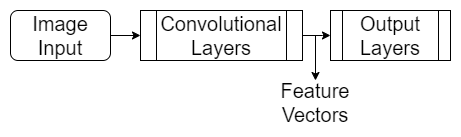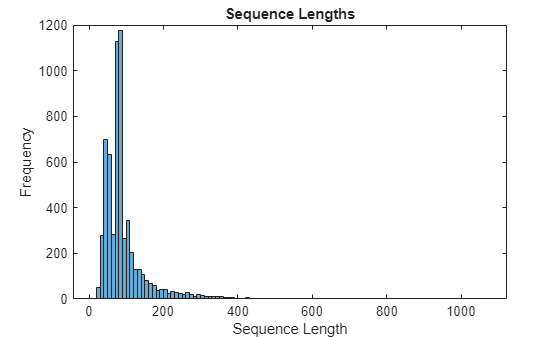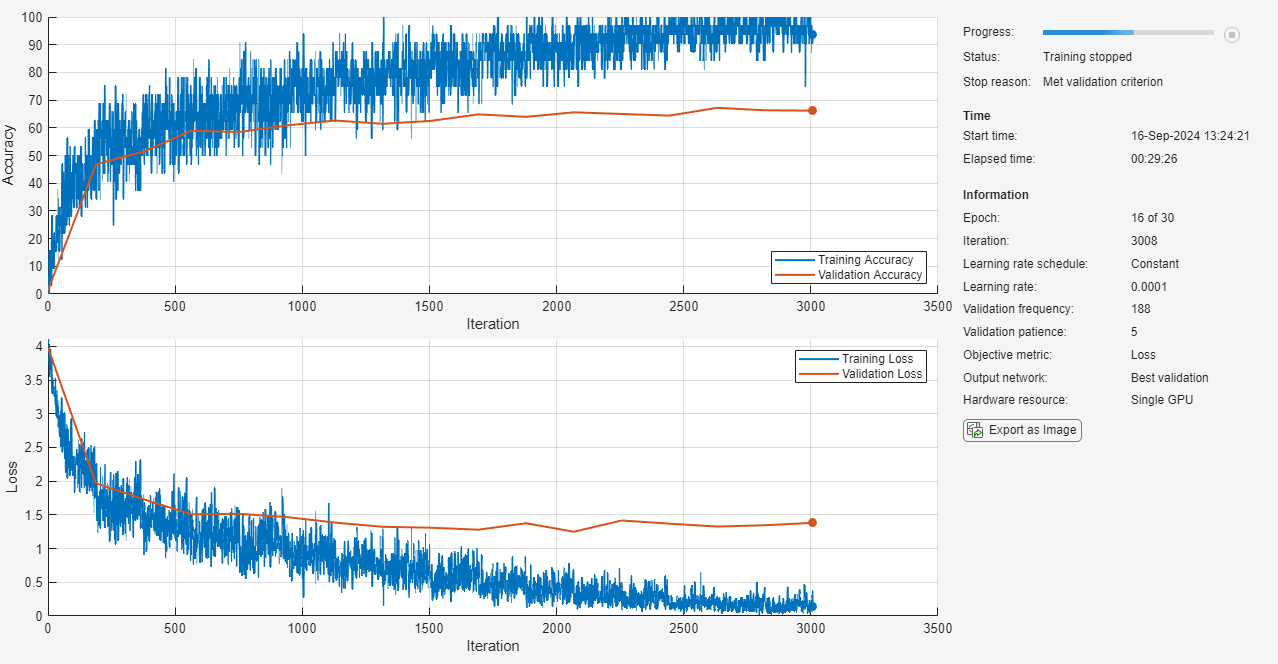Documentation

### This is machine translation

Mouseover text to see original. Click the button below to return to the English version of the page.

Note: This page has been translated by MathWorks. Click here to see
To view all translated materials including this page, select Country from the country navigator on the bottom of this page.

## Classify Videos Using Deep Learning

This example shows how to create a network for video classification by combining a pretrained image classification model and an LSTM network.

To create a deep learning network for video classification:

1. Convert videos to sequences of feature vectors using a pretrained convolutional neural network, such as GoogLeNet, to extract features from each frame.

2. Train an LSTM network on the sequences to predict the video labels.

3. Assemble a network that classifies videos directly by combining layers from both networks.

The following diagram illustrates the network architecture.

• To input image sequences to the network, use a sequence input layer.

• To use convolutional layers to extract features, that is, to apply the convolutional operations to each frame of the videos independently, use a sequence folding layer followed by the convolutional layers.

• To restore the sequence structure and reshape the output to vector sequences, use a sequence unfolding layer and a flatten layer.

• To classify the resulting vector sequences, include the LSTM layers followed by the output layers.### Load Pretrained Convolutional Network

To convert frames of videos to feature vectors, use the activations of a pretrained network.

Load a pretrained GoogLeNet model using the `googlenet` function. This function requires the Deep Learning Toolbox™ Model for GoogLeNet Network support package. If this support package is not installed, then the function provides a download link.

`netCNN = googlenet;`

### Load Data

Download the HBMD51 data set from HMDB: a large human motion database and extract the RAR file into a folder named `"hmdb51_org"`. The data set contains about 2 GB of video data for 7000 clips over 51 classes, such as `"drink"`, `"run"`, and `"shake_hands"`.

After extracting the RAR files, use the supporting function `hmdb51Files` to get the file names and the labels of the videos.

```dataFolder = "hmdb51_org"; [files,labels] = hmdb51Files(dataFolder);```

Read the first video using the `readVideo` helper function, defined at the end of this example, and view the size of the video. The video is a H-by-W-by-C-by-S array, where H, W, C, and S are the height, width, number of channels, and number of frames of the video, respectively.

```idx = 1; filename = files(idx); video = readVideo(filename); size(video)```
```ans = 1×4 240 320 3 409 ```

View the corresponding label.

`labels(idx)`
```ans = categorical brush_hair ```

To view the video, use the `implay` function (requires Image Processing Toolbox™). This function expects data in the range [0,1], so you must first divide the data by 255. Alternatively, you can loop over the individual frames and use the `imshow` function.

```numFrames = size(video,4); figure for i = 1:numFrames frame = video(:,:,:,i); imshow(frame/255); drawnow end```

### Convert Frames to Feature Vectors

Use the convolutional network as a feature extractor by getting the activations when inputting the video frames to the network. Convert the videos to sequences of feature vectors, where the feature vectors are the output of the `activations` function on the last pooling layer of the GoogLeNet network (`"pool5-7x7_s1"`).

This diagram illustrates the data flow through the network.To read the video data and resize it to match the input size of the GoogLeNet network, use the `readVideo` and `centerCrop` helper functions, defined at the end of this example. This step can take a long time to run. After converting the videos to sequences, save the sequences in a MAT-file in the `tempdir` folder. If the MAT file already exists, then load the sequences from the MAT-file without reconverting them.

```inputSize = netCNN.Layers(1).InputSize(1:2); layerName = "pool5-7x7_s1"; tempFile = fullfile(tempdir,"hmdb51_org.mat"); if exist(tempFile,'file') load(tempFile,"sequences") else numFiles = numel(files); sequences = cell(numFiles,1); for i = 1:numFiles fprintf("Reading file %d of %d...\n", i, numFiles) video = readVideo(files(i)); video = centerCrop(video,inputSize); sequences{i,1} = activations(netCNN,video,layerName,'OutputAs','columns'); end save(tempFile,"sequences","-v7.3"); end```

View the sizes of the first few sequences. Each sequence is a D-by-S array, where D is the number of features (the output size of the pooling layer) and S is the number of frames of the video.

`sequences(1:10)`
```ans = 10×1 cell array {1024×409 single} {1024×395 single} {1024×323 single} {1024×246 single} {1024×159 single} {1024×137 single} {1024×359 single} {1024×191 single} {1024×439 single} {1024×528 single} ```

### Prepare Training Data

Prepare the data for training by partitioning the data into training and validation partitions and removing any long sequences.

Create Training and Validation Partitions

Partition the data. Assign 90% of the data to the training partition and 10% to the validation partition.

```numObservations = numel(sequences); idx = randperm(numObservations); N = floor(0.9 * numObservations); idxTrain = idx(1:N); sequencesTrain = sequences(idxTrain); labelsTrain = labels(idxTrain); idxValidation = idx(N+1:end); sequencesValidation = sequences(idxValidation); labelsValidation = labels(idxValidation);```

Remove Long Sequences

Sequences that are much longer than typical sequences in the networks can introduce lots of padding into the training process. Having too much padding can negatively impact the classification accuracy.

Get the sequence lengths of the training data and visualize them in a histogram of the training data.

```numObservationsTrain = numel(sequencesTrain); sequenceLengths = zeros(1,numObservationsTrain); for i = 1:numObservationsTrain sequence = sequencesTrain{i}; sequenceLengths(i) = size(sequence,2); end figure histogram(sequenceLengths) title("Sequence Lengths") xlabel("Sequence Length") ylabel("Frequency")```Only a few sequences have more than 400 time steps. To improve the classification accuracy, remove the training sequences that have more than 400 time steps along with their corresponding labels.

```maxLength = 400; idx = sequenceLengths > maxLength; sequencesTrain(idx) = []; labelsTrain(idx) = [];```

### Create LSTM Network

Next, create an LSTM network that can classify the sequences of feature vectors representing the videos.

Define the LSTM network architecture. Specify the following network layers.

• A sequence input layer with an input size corresponding to the feature dimension of the feature vectors

• A BiLSTM layer with 2000 hidden units with a dropout layer afterwards. To output only one label for each sequence by setting the `'OutputMode'` option of the BiLSTM layer to `'last'`

• A fully connected layer with an output size corresponding to the number of classes, a softmax layer, and a classification layer.

```numFeatures = size(sequencesTrain{1},1); numClasses = numel(categories(labelsTrain)); layers = [ sequenceInputLayer(numFeatures,'Name','sequence') bilstmLayer(2000,'OutputMode','last','Name','bilstm') dropoutLayer(0.5,'Name','drop') fullyConnectedLayer(numClasses,'Name','fc') softmaxLayer('Name','softmax') classificationLayer('Name','classification')];```

### Specify Training Options

Specify the training options using the `trainingOptions` function.

• Set a mini-batch size 16, an initial learning rate of 0.0001, and a gradient threshold of 2 (to prevent the gradients from exploding).

• Truncate the sequences in each mini-batch to have the same length as the shortest sequence.

• Shuffle the data every epoch.

• Validate the network once per epoch.

• Display the training progress in a plot and suppress verbose output.

```miniBatchSize = 16; numObservations = numel(sequencesTrain); numIterationsPerEpoch = floor(numObservations / miniBatchSize); options = trainingOptions('adam', ... 'MiniBatchSize',miniBatchSize, ... 'InitialLearnRate',1e-4, ... 'GradientThreshold',2, ... 'Shuffle','every-epoch', ... 'ValidationData',{sequencesValidation,labelsValidation}, ... 'ValidationFrequency',numIterationsPerEpoch, ... 'Plots','training-progress', ... 'Verbose',false);```

### Train LSTM Network

Train the network using the `trainNetwork` function. This can take a long time to run.

`[netLSTM,info] = trainNetwork(sequencesTrain,labelsTrain,layers,options);`Calculate the classification accuracy of the network on the validation set. Use the same mini-batch size as for the training options.

```YPred = classify(netLSTM,sequencesValidation,'MiniBatchSize',miniBatchSize); YValidation = labelsValidation; accuracy = mean(YPred == YValidation)```
```accuracy = 0.6647 ```

### Assemble Video Classification Network

To create a network that classifies videos directly, assemble a network using layers from both of the created networks. Use the layers from the convolutional network to transform the videos into vector sequences and the layers from the LSTM network to classify the vector sequences.

The following diagram illustrates the network architecture.

• To input image sequences to the network, use a sequence input layer.

• To use convolutional layers to extract features, that is, to apply the convolutional operations to each frame of the videos independently, use a sequence folding layer followed by the convolutional layers.

• To restore the sequence structure and reshape the output to vector sequences, use a sequence unfolding layer and a flatten layer.

• To classify the resulting vector sequences, include the LSTM layers followed by the output layers.Add Convolutional Layers

First, create a layer graph of the GoogLeNet network.

`cnnLayers = layerGraph(netCNN);`

Remove the input layer (`"data"`) and the layers after the pooling layer used for the activations (`"pool5-drop_7x7_s1"`, `"loss3-classifier"`, `"prob"`, and `"output"`).

```layerNames = ["data" "pool5-drop_7x7_s1" "loss3-classifier" "prob" "output"]; cnnLayers = removeLayers(cnnLayers,layerNames);```

Add Sequence Input Layer

Create a sequence input layer that accepts image sequences containing images of the same input size as the GoogLeNet network. To normalize the images using the same average image as the GoogLeNet network, set the `'Normalization'` option of the sequence input layer to `'zerocenter'` and the `'Mean'` option to the average image of the input layer of GoogLeNet.

```inputSize = netCNN.Layers(1).InputSize(1:2); averageImage = netCNN.Layers(1).AverageImage; inputLayer = sequenceInputLayer([inputSize 3], ... 'Normalization','zerocenter', ... 'Mean',averageImage, ... 'Name','input');```

Add the sequence input layer to the layer graph. To apply the convolutional layers to the images of the sequences independently, remove the sequence structure of the image sequences by including a sequence folding layer between the sequence input layer and the convolutional layers. Connect the output of the sequence folding layer to the input of the first convolutional layer (`"conv1-7x7_s2"`).

```layers = [ inputLayer sequenceFoldingLayer('Name','fold')]; lgraph = addLayers(cnnLayers,layers); lgraph = connectLayers(lgraph,"fold/out","conv1-7x7_s2");```

Add LSTM Layers

Add the LSTM layers to the layer graph by removing the sequence input layer of the LSTM network. To restore the sequence structure removed by the sequence folding layer, include a sequence unfolding layer after the convolution layers. The LSTM layers expect sequences of vectors. To reshape the output of the sequence unfolding layer to vector sequences, include a flatten layer after the sequence unfolding layer.

Take the layers from the LSTM network and remove the sequence input layer.

```lstmLayers = netLSTM.Layers; lstmLayers(1) = [];```

Add the sequence folding layer, the flatten layer, and the LSTM layers to the layer graph. Connect the last convolutional layer (`"pool5-7x7_s1"`) to the input of the sequence unfolding layer (`"unfold/in")`.

```layers = [ sequenceUnfoldingLayer('Name','unfold') flattenLayer('Name','flatten') lstmLayers]; lgraph = addLayers(lgraph,layers); lgraph = connectLayers(lgraph,"pool5-7x7_s1","unfold/in");```

To enable the unfolding layer to restore the sequence structure, connect the `"miniBatchSize"` output of the sequence folding layer to the corresponding input of the sequence unfolding layer.

`lgraph = connectLayers(lgraph,"fold/miniBatchSize","unfold/miniBatchSize");`

Assemble Network

Check that the network is valid using the `analyzeNetwork` function.

`analyzeNetwork(lgraph)`

Assemble the network so that it is ready for prediction using the `assembleNetwork` function.

`net = assembleNetwork(lgraph)`
```net = DAGNetwork with properties: Layers: [148×1 nnet.cnn.layer.Layer] Connections: [175×2 table] ```

### Classify Using New Data

Read and center-crop the video `"pushup.mp4"` using the same steps as before.

```filename = "pushup.mp4"; video = readVideo(filename);```

To view the video, use the `implay` function (requires Image Processing Toolbox). This function expects data in the range [0,1], so you must first divide the data by 255. Alternatively, you can loop over the individual frames and use the `imshow` function.

```numFrames = size(video,4); figure for i = 1:numFrames frame = video(:,:,:,i); imshow(frame/255); drawnow end```Classify the video using the assembled network. The `classify` function expects a cell array containing the input videos, so you must input a 1-by-1 cell array containing the video.

```video = centerCrop(video,inputSize); YPred = classify(net,{video})```
```YPred = categorical pushup ```

### Helper Functions

The `readVideo` function reads the video in `filename` and returns an `H`-by-`W`-by-`C-`by-`S` array, where `H`, `W`, `C`, and `S` are the height, width, number of channels, and number of frames of the video, respectively.

```function video = readVideo(filename) vr = VideoReader(filename); H = vr.Height; W = vr.Width; C = 3; % Preallocate video array numFrames = floor(vr.Duration * vr.FrameRate); video = zeros(H,W,C,numFrames); % Read frames i = 0; while hasFrame(vr) i = i + 1; video(:,:,:,i) = readFrame(vr); end % Remove unallocated frames if size(video,4) > i video(:,:,:,i+1:end) = []; end end```

The `centerCrop` function crops the longest edges of a video and resizes it have size `inputSize`.

```function videoResized = centerCrop(video,inputSize) sz = size(video); if sz(1) < sz(2) % Video is landscape idx = floor((sz(2) - sz(1))/2); video(:,1:(idx-1),:,:) = []; video(:,(sz(1)+1):end,:,:) = []; elseif sz(2) < sz(1) % Video is portrait idx = floor((sz(1) - sz(2))/2); video(1:(idx-1),:,:,:) = []; video((sz(2)+1):end,:,:,:) = []; end videoResized = imresize(video,inputSize(1:2)); end```

Download ebook